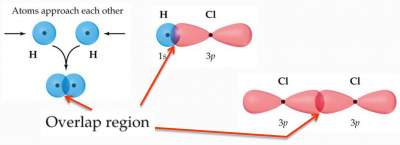# Covalent Bonding and Orbital Overlap

1. Does not explain why a bond forms.
2. Does not tell which orbitals are involved in bonding?
Hence, the need of Valence Bond Theory where "Bonds form when orbitals on atoms overlap".

• As two atoms approach each other their atomic orbitals overlap.
• As the amount of overlap increases, the energy of the interaction decreases.
• At some distance the minimum energy is reached. The minimum energy corresponds to the bonding distance (bond length).
• At some distance the minimum energy is reached. The minimum energy corresponds to the bonding distance (bond length).
• As the two atoms get closer, their nuclei begin to repel and the energy increases.

### Example: Overalp of orbitals explains the mechanism of bond formation